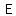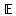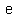# U+1D53C MATHEMATICAL DOUBLE-STRUCK CAPITAL E

U+1D53C was added to Unicode in version 3.1 (2001). It belongs to the blockMathematical Alphanumeric Symbols in the Supplementary Multilingual Plane.

This character is a Uppercase Letter and is commonly used, that is, in no specific script.

The glyph is a Font composition of the glyphs. It has a Neutral East Asian Width. In bidirectional context it acts as Left To Right and is not mirrored. The glyph can, under circumstances, be confused with 38 other glyphs. In text U+1D53C behaves as Alphabetic regarding line breaks. It has type Upper for sentence and ALetter for word breaks. The Grapheme Cluster Break is Any.

In probability theory, the expected value of a random variable is intuitively the long-run average value of repetitions of the experiment it represents. For example, the expected value of a dice roll is 3.5 because, roughly speaking, the average of an extremely large number of dice rolls is practically always nearly equal to 3.5. Less roughly, the law of large numbers guarantees that the arithmetic mean of the values almost surely converges to the expected value as the number of repetitions goes to infinity. The expected value is also known as the expectation, mathematical expectation, EV, mean, or first moment.

More practically, the expected value of a discrete random variable is the probability-weighted average of all possible values. In other words, each possible value the random variable can assume is multiplied by its probability of occurring, and the resulting products are summed to produce the expected value. The same works for continuous random variables, except the sum is replaced by an integral and the probabilities by probability densities. The formal definition subsumes both of these and also works for distributions which are neither discrete nor continuous: the expected value of a random variable is the integral of the random variable with respect to its probability measure.

The expected value does not exist for random variables having some distributions with large "tails", such as the Cauchy distribution. For random variables such as these, the long-tails of the distribution prevent the sum/integral from converging.

The expected value is a key aspect of how one characterizes a probability distribution; it is one type of location parameter. By contrast, the variance is a measure of dispersion of the possible values of the random variable around the expected value. The variance itself is defined in terms of two expectations: it is the expected value of the squared deviation of the variable's value from the variable's expected value.

The expected value plays important roles in a variety of contexts. In regression analysis, one desires a formula in terms of observed data that will give a "good" estimate of the parameter giving the effect of some explanatory variable upon a dependent variable. The formula will give different estimates using different samples of data, so the estimate it gives is itself a random variable. A formula is typically considered good in this context if it is an unbiased estimator—that is, if the expected value of the estimate (the average value it would give over an arbitrarily large number of separate samples) can be shown to equal the true value of the desired parameter.

In decision theory, and in particular in choice under uncertainty, an agent is described as making an optimal choice in the context of incomplete information. For risk neutral agents, the choice involves using the expected values of uncertain quantities, while for risk averse agents it involves maximizing the expected value of some objective function such as a von Neumann-Morgenstern utility function. One example of using expected value in reaching optimal decisions is the Gordon-Loeb Model of information security investment. According to the model, one can conclude that the amount a firm spends to protect information should generally be only a small fraction of the expected loss (i.e., the expected value of the loss resulting from a cyber/information security breach).

## Representations

System Representation
120124
UTF-8 F0 9D 94 BC
UTF-16 D8 35 DD 3C
UTF-32 00 01 D5 3C
URL-Quoted %F0%9D%94%BC
HTML-Escape &#x1D53C;
Wrong windows-1252 Mojibake ð¼
HTML-Escape &Eopf;
LaTeX \mathbb{E}

## Complete Record

Property Value
Age (age) 3.1
Unicode Name (na) MATHEMATICAL DOUBLE-STRUCK CAPITAL E
Unicode 1 Name (na1)
Block (blk) Math_Alphanum
General Category (gc) Uppercase Letter
Script (sc) Common
Bidirectional Category (bc) Left To Right
Combining Class (ccc) Not Reordered
Decomposition Type (dt) Font
Decomposition Mapping (dm)Lowercase (Lower)
Simple Lowercase Mapping (slc)Lowercase Mapping (lc)Uppercase (Upper)
Simple Uppercase Mapping (suc)Uppercase Mapping (uc)Simple Titlecase Mapping (stc)Titlecase Mapping (tc)Case Folding (cf)ASCII Hex Digit (AHex)
Alphabetic (Alpha)
Bidi Control (Bidi_C)
Bidi Mirrored (Bidi_M)
Bidi Paired Bracket (bpb)Bidi Paired Bracket Type (bpt) None
Cased (Cased)
Composition Exclusion (CE)
Case Ignorable (CI)
Full Composition Exclusion (Comp_Ex)
Changes When Casefolded (CWCF)
Changes When Casemapped (CWCM)
Changes When NFKC Casefolded (CWKCF)
Changes When Lowercased (CWL)
Changes When Titlecased (CWT)
Changes When Uppercased (CWU)
Dash (Dash)
Deprecated (Dep)
Default Ignorable Code Point (DI)
Diacritic (Dia)
East Asian Width (ea) Neutral
Extender (Ext)
FC NFKC Closure (FC_NFKC)Grapheme Cluster Break (GCB) Any
Grapheme Base (Gr_Base)
Grapheme Extend (Gr_Ext)
Hex Digit (Hex)
Hangul Syllable Type (hst) Not Applicable
Hyphen (Hyphen)
ID Continue (IDC)
Ideographic (Ideo)
ID Start (IDS)
IDS Binary Operator (IDSB)
IDS Trinary Operator and (IDST)
InMC (InMC)
Indic Positional Category (InPC) NA
Indic Syllabic Category (InSC) Other
ISO 10646 Comment (isc)
Joining Group (jg) No_Joining_Group
Join Control (Join_C)
Jamo Short Name (JSN)
Joining Type (jt) Non Joining
Line Break (lb) Alphabetic
Logical Order Exception (LOE)
Math (Math)
Noncharacter Code Point (NChar)
NFC Quick Check (NFC_QC) Yes
NFD Quick Check (NFD_QC) Yes
NFKC Casefold (NFKC_CF)NFKC Quick Check (NFKC_QC) No
NFKD Quick Check (NFKD_QC) No
Numeric Type (nt) None
Numeric Value (nv) NaN
Other Alphabetic (OAlpha)
Other Default Ignorable Code Point (ODI)
Other Grapheme Extend (OGr_Ext)
Other ID Continue (OIDC)
Other ID Start (OIDS)
Other Lowercase (OLower)
Other Math (OMath)
Other Uppercase (OUpper)
Pattern Syntax (Pat_Syn)
Pattern White Space (Pat_WS)
Quotation Mark (QMark)
Sentence Break (SB) Upper
Simple Case Folding (scf)Script Extension (scx) Common
Soft Dotted (SD)
STerm (STerm)
Terminal Punctuation (Term)
Unified Ideograph (UIdeo)
Variation Selector (VS)
Word Break (WB) ALetter
White Space (WSpace)
XID Continue (XIDC)
XID Start (XIDS)
Expands On NFC (XO_NFC)
Expands On NFD (XO_NFD)
Expands On NFKC (XO_NFKC)
Expands On NFKD (XO_NFKD)